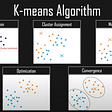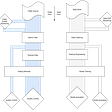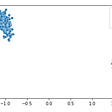# PyTorch vs Tensorflow (Deep Learning Model)

`data.shape:(1368, 5)`

## Train Test Split:

`print ('Training Set: %d, Test Set: %d \n' % (len(x_train), len(x_test)))Training Set: 957, Test Set: 411`

Installing PyTorch and Tensorflow:

2. !pip install — upgrade tensorflow

1. PyTorch:
`import torchimport torch.nn as nnimport torch.utils.data as td# Set random seed for reproducabilitytorch.manual_seed(0)print("Libraries imported - ready to use PyTorch", torch.__version__)Libraries imported - ready to use PyTorch 1.7.1+cpu`
`import tensorflowfrom tensorflow import kerasfrom tensorflow.keras import modelsfrom tensorflow.keras.models import Sequentialfrom tensorflow.keras.layers import Densefrom tensorflow.keras import utilsfrom tensorflow.keras import optimizers# Set random seed for reproducabilitytensorflow.random.set_seed(0)print("Libraries imported.")print('Keras version:',keras.__version__)print('TensorFlow version:',tensorflow.__version__)Libraries imported.Keras version: 2.2.4-tfTensorFlow version: 2.0.0-alpha0`

PyTorch:

`# Preparing Train datatrain_x = torch.Tensor(x_train).float()train_y = torch.Tensor(y_train).long()train_ds = td.TensorDataset(train_x, train_y)train_loader = td.DataLoader(train_ds, batch_size=20, shuffle=False, num_workers=1)# Preparing Test datatest_x = torch.Tensor(x_test).float()test_y = torch.Tensor(y_test).long()test_ds = td.TensorDataset(test_x, test_y)test_loader = td.DataLoader(test_ds, batch_size=20, shuffle=False, num_workers=1)`

Tensorflow:

`# Set data types for float featuresx_train = x_train.astype('float32')x_test = x_test.astype('float32')# Set data types for categorical labelsy_train = utils.to_categorical(y_train)y_test = utils.to_categorical(y_test)`

PyTorch:

`class NModel(nn.Module):    def __init__(self):        super(NModel, self).__init__()        self.fc1 = nn.Linear(len(features), 10)        self.fc2 = nn.Linear(10, 10)        self.fc3 = nn.Linear(10, 3)            def forward(self, x):        x = torch.relu(self.fc1(x))        x = torch.relu(self.fc2(x))        x = torch.softmax(self.fc3(x), dim=1)                return xmodel = NModel()print(model)Output:NModel(  (fc1): Linear(in_features=4, out_features=10, bias=True)  (fc2): Linear(in_features=10, out_features=10, bias=True)  (fc3): Linear(in_features=10, out_features=3, bias=True))`

Tensorflow:

`model = Sequential()model.add(Dense(10, input_dim=len(features), activation='relu'))model.add(Dense(10, input_dim=10, activation='relu'))model.add(Dense(len(penguin_classes), input_dim=10, activation='softmax'))print(model.summary())Model: "sequential"_________________________________________________________________Layer (type)                 Output Shape              Param #   =================================================================dense (Dense)                (None, 10)                50        _________________________________________________________________dense_1 (Dense)              (None, 10)                110       _________________________________________________________________dense_2 (Dense)              (None, 3)                 33        =================================================================Total params: 193Trainable params: 193Non-trainable params: 0_________________________________________________________________None`

PyTorch:

1. Train:
`def train(model, data_loader, optimizer):    # Set the model to training mode    model.train()    train_loss = 0        for batch, tensor in enumerate(data_loader):        data, target = tensor        # feedforward step        optimizer.zero_grad()        out = model(data)        loss = loss_criteria(out, target)        train_loss += loss.item()        # backpropagation to improve the model performances        loss.backward()        optimizer.step()    # Return average loss of the training     avg_loss = train_loss / (batch+1)    print('Training set: Average loss: {:.6f}'.format(avg_loss))    return avg_loss`
`def test(model, data_loader):    model.eval()    test_loss = 0    correct = 0with torch.no_grad():        batch_count = 0        for batch, tensor in enumerate(data_loader):            batch_count += 1            data, target = tensor            # Get the predictions            out = model(data)            # calculate the loss            test_loss += loss_criteria(out, target).item()            # Calculate the accuracy            _, predicted = torch.max(out.data, 1)            correct += torch.sum(target==predicted).item()                # Calculate the average loss and total accuracy for this epoch    avg_loss = test_loss/batch_count    print('Validation set: Average loss: {:.6f}, Accuracy: {}/{} ({:.0f}%)\n'.format(        avg_loss, correct, len(data_loader.dataset),        100. * correct / len(data_loader.dataset)))        # return average loss for the epoch    return avg_loss`
`# Specify the loss criteria (CrossEntropyLoss for multi-class classification)loss_criteria = nn.CrossEntropyLoss()# We are using Adam optimizerlearning_rate = 0.001 # try different learning rateoptimizer = torch.optim.Adam(model.parameters(), lr=learning_rate) # setting the Adam algorithmoptimizer.zero_grad()# We'll be saving metrics for each epoch in these arraysepoch_nums = []training_loss = []validation_loss = []# Train over 50 epochsepochs = 50for epoch in range(1, epochs + 1):    print('Epoch: {}'.format(epoch))        # Feed training data into the model to optimize the weights    train_loss = train(model, train_loader, optimizer)        # Feed the test data into the model to check its performance    test_loss = test(model, test_loader)        # Log the metrics for this epoch    epoch_nums.append(epoch)    training_loss.append(train_loss)    validation_loss.append(test_loss)`
`Epoch: 47Training set: Average loss: 0.569989Validation set: Average loss: 0.572491, Accuracy: 408/411 (99%)Epoch: 48Training set: Average loss: 0.569646Validation set: Average loss: 0.572059, Accuracy: 408/411 (99%)Epoch: 49Training set: Average loss: 0.569319Validation set: Average loss: 0.571643, Accuracy: 408/411 (99%)Epoch: 50Training set: Average loss: 0.569007Validation set: Average loss: 0.571244, Accuracy: 408/411 (99%)`

Tensorflow:

1. Train and Test:
`learning_rate = 0.001 # learning rateopt = optimizers.Adam(lr=learning_rate) # model optimizer# compiling the model with CC loss and optimizer! Along with Acc. metrics!model.compile(loss='categorical_crossentropy',              optimizer=opt,              metrics=['accuracy'])# we will do 50 sets of run and in epochs, 10 will be the batch sizenum_epochs = 50history = model.fit(x_train, y_train, epochs=num_epochs, batch_size=10, validation_data=(x_test, y_test))`
`Epoch 47/50957/957 [==============================] - ETA: 0s - loss: 0.1854 - accuracy: 0.90 - ETA: 0s - loss: 0.1081 - accuracy: 0.97 - 0s 109us/sample - loss: 0.1073 - accuracy: 0.9739 - val_loss: 0.1230 - val_accuracy: 0.9830Epoch 48/50957/957 [==============================] - ETA: 0s - loss: 0.0697 - accuracy: 1.00 - ETA: 0s - loss: 0.1037 - accuracy: 0.97 - 0s 116us/sample - loss: 0.0994 - accuracy: 0.9812 - val_loss: 0.1116 - val_accuracy: 0.9805Epoch 49/50957/957 [==============================] - ETA: 0s - loss: 0.0896 - accuracy: 1.00 - ETA: 0s - loss: 0.1031 - accuracy: 0.97 - 0s 122us/sample - loss: 0.0968 - accuracy: 0.9770 - val_loss: 0.1008 - val_accuracy: 0.9830Epoch 50/50957/957 [==============================] - ETA: 0s - loss: 0.0447 - accuracy: 1.00 - ETA: 0s - loss: 0.0942 - accuracy: 0.97 - 0s 126us/sample - loss: 0.0922 - accuracy: 0.9833 - val_loss: 0.1066 - val_accuracy: 0.9781`

Visualize the training and validation Phase:

`%matplotlib inlinefrom matplotlib import pyplot as pltepoch_nums = range(1,num_epochs+1)training_loss = history.history["loss"]validation_loss = history.history["val_loss"]plt.plot(epoch_nums, training_loss)plt.plot(epoch_nums, validation_loss)plt.xlabel('epoch')plt.ylabel('loss')plt.legend(['training', 'validation'], loc='upper right')plt.show()`

## Model Weights and Biases:

PyTorch:

`fc1.weight  [[-0.00374341  0.2682218  -0.41152257 -0.3679695 ] [-0.20487966 -0.28398493  0.13597898  0.6053104 ] [-0.04437202  0.13230628 -0.15110654 -0.09828269] [-0.47767425 -0.33114105 -0.20611155  0.01852179] [ 0.17907079  0.28123733 -0.35748705 -0.23587279] [ 0.40088734  0.382148   -0.20181231  0.3537034 ] [-0.08059168  0.05290705  0.4527381  -0.46383518] [-0.35555413 -0.16605727 -0.23530953  0.38971528] [-0.32408983 -0.23016644 -0.34932023 -0.4682805 ] [-0.43653095  0.80248505  0.29239044  0.17577983]]fc1.bias  [ 0.02629578 -0.26949045  0.08459234 -0.46684736 -0.3798124  -0.4262236  0.31546897  0.25337356 -0.22174752  0.2345465 ]fc2.weight  [[ 2.02246875e-01  3.14372510e-01  1.25505149e-01  4.27201092e-02   2.12026387e-01 -1.86195642e-01  5.89271486e-02 -2.45173126e-01  -2.19173074e-01 -1.63358063e-01] [ 1.43084526e-01  1.60798699e-01 -1.87318310e-01  9.55346525e-02   1.92188621e-01  1.52368024e-01  1.20740533e-02  4.16618437e-02   1.96180314e-01  9.25334394e-01] [-2.43692577e-01 -1.43560484e-01  1.24280632e-01  2.62010306e-01   2.59306699e-01  3.23746145e-01  6.29339218e-02 -2.45525643e-01   2.90905833e-02 -6.68823123e-01] [-2.94709772e-01  5.11151612e-01  2.40446895e-01 -3.15446049e-01   5.91889918e-02 -1.04206558e-02 -5.20388186e-02 -1.01968892e-01   1.21607333e-01 -4.82648820e-01] [ 1.15926355e-01  1.59918934e-01  2.26378471e-01  1.18241072e-01  -3.12981755e-01 -2.05135971e-01  1.57897264e-01  6.61869049e-02  -2.46684223e-01 -1.82090104e-01] [ 2.97491044e-01  5.08512676e-01 -1.37883261e-01 -7.95897096e-02  -3.19968253e-01 -9.41922441e-02 -2.38138139e-01 -2.13026911e-01  -1.74240172e-02 -3.14111978e-01] [-1.29504845e-01  1.87642485e-01 -1.92436963e-01  2.86935598e-01   2.16710836e-01 -2.66669482e-01 -7.87041336e-02  1.42690241e-02   4.61379588e-02  7.50010908e-02] [ 1.24096721e-01  1.89420879e-02 -1.54296622e-01  1.49635494e-01  -3.03341120e-01 -1.87430307e-01 -7.91612566e-02 -1.54038772e-01  -1.10627025e-01 -2.59187132e-01] [-6.72664344e-02  3.37419212e-01 -2.06011564e-01 -1.62286162e-02   2.08991051e-01 -1.28818750e-01  8.78867507e-03  8.23016744e-04   6.39986098e-02  2.39946589e-01] [ 2.99545556e-01  2.00822324e-01  3.00230891e-01 -2.28701234e-02  -2.84074187e-01 -1.49916381e-01  2.15321153e-01 -2.04995275e-03  -1.57179862e-01 -2.42329061e-01]]fc2.bias  [-0.2959424   0.13159421 -0.27384382  0.07626942  0.17096573 -0.4653062  0.19725719 -0.24745122 -0.09499434 -0.1282217 ]fc3.weight  [[-0.06091028  0.32780746 -0.9960096  -1.0807507  -0.04948315 -0.3577183  -0.14365433  0.11912274  0.19421846 -0.02134135] [ 0.27809682 -0.47728527  0.09838016  0.83235973 -0.2853832   0.9088981  -0.03649095 -0.14116624  0.38886335 -0.25554216] [ 0.03393281 -0.19362502  1.0301037  -0.24135342  0.15194914 -0.6721673  -0.07604478 -0.06650442 -1.1390747   0.17134616]]fc3.bias  [ 0.5242965  -0.09666859 -0.13340557]`

Tensorflow:

`------------Weights: [[-0.27236846 -0.3841947   0.03234848  0.08020484 -0.10909867  0.06330433  -0.19313203  0.84640336  0.36701852 -0.4905011 ] [ 0.27471453  0.21265197  0.08079417 -0.17707926 -0.10406601  0.80510986   0.3481228  -0.04539559 -0.62267196 -0.5447268 ] [-0.28836262 -0.634329    0.28234363  0.34767175  0.23550075 -0.00817027   0.14570452 -0.7969777  -0.5233428   0.3296095 ] [-0.42851955 -0.24623463 -0.2871196  -0.5230521  -0.43773973  0.37682083  -0.0763066   0.23378253  0.74960405 -0.4691702 ]] Biases: [ 0.          0.         -0.01398832  0.          0.          0.20292243 -0.19176428 -0.2576086  -0.3294029   0.        ]------------Weights: [[ 0.0607031  -0.30530828  0.39975524  0.3037489   0.15896738  0.03326017  -0.53190327  0.40915883 -0.03316814 -0.1240823 ] [ 0.42301047  0.14984506 -0.54566675  0.3919103  -0.4295466   0.50397205  -0.31616646  0.17803025 -0.41518384 -0.38429344] [ 0.5336163   0.37752342 -0.46671256  0.17206895 -0.04215616  0.5297911   0.4396385   0.2792814   0.2627702  -0.2233491 ] [-0.04491103  0.19579428 -0.26655364  0.17358297  0.3112036   0.53520477  -0.3109483  -0.5284722  -0.00098199 -0.44063687] [ 0.5135      0.39074183  0.39206952 -0.03048635  0.02663547  0.20555359   0.09307003  0.24590033 -0.49007446 -0.2917699 ] [ 0.50351286 -0.3901862   0.6034433   0.29417115 -0.26569188 -0.5313456   0.41638133 -0.15550071  0.00485109 -0.04526619] [ 0.26545775 -0.19090915  0.06236434 -0.30627972  0.12806404 -0.38203925  -0.21348043  0.41777134  0.26362625 -0.51192576] [-0.43856043 -0.21495155 -0.1461127  -0.46094683 -0.20800981  0.3025053  -0.00639509  0.40292972  0.2737926  -0.2580092 ] [-0.5078605  -0.4176368  -0.24811995 -0.49696004 -0.27225545 -0.38996994  -0.4037082   0.16782355 -0.2606465   0.22881041] [ 0.32320213 -0.30822456 -0.37115166  0.45703936 -0.35191107  0.24120325  -0.2000556   0.23292273 -0.33508268 -0.51532805]] Biases: [-0.03098752  0.          0.44007653  0.          0.          0.  0.13158555 -0.28722948 -0.30139711 -0.01467387]------------Weights: [[-0.38429576  0.3262583  -0.04640121] [ 0.50995994 -0.12620813 -0.6595991 ] [ 0.54413134  0.01471629 -0.22374861] [ 0.54463303 -0.50025463  0.06109887] [ 0.26757038 -0.67376095 -0.18467396] [ 0.08888024 -0.2536324   0.20257705] [ 1.1826342  -1.5543535   0.6450739 ] [-0.48484278  0.38147986  0.19515398] [-0.51660526 -0.35239688  0.6803643 ] [ 0.6415732  -0.61359274 -0.03051702]] Biases: [ 0.3130718   0.48137134 -0.49553102]`

## Confusion Matrix:

PyTorch and Tensorflow:

`import numpy as npfrom sklearn.metrics import confusion_matriximport matplotlib.pyplot as plt%matplotlib inlineclass_probabilities = model.predict(x_test)predictions = np.argmax(class_probabilities, axis=1)true_labels = np.argmax(y_test, axis=1)# Plot the confusion matrixcm = confusion_matrix(true_labels, predictions)plt.imshow(cm, interpolation="nearest", cmap=plt.cm.Blues)plt.colorbar()tick_marks = np.arange(len(penguin_classes))plt.xticks(tick_marks, penguin_classes, rotation=85)plt.yticks(tick_marks, penguin_classes)plt.xlabel("Actual Class")plt.ylabel("Predicted Class")plt.show()`

--

--

--

## More from Mahedi Hasan Jisan

Python Developer

Love podcasts or audiobooks? Learn on the go with our new app.

## How to Use K-Means Algorithm to Create Customer Segmentation Tools## INTRODUCTION TO MACHINE LEARNING PIPELINES## Why I’m getting a (nano)degree in Deep Learning## How We Generated Price Predictions## Imputing Missing values## How To Discover The Laws Of Physics With Deep Learning and Symbolic Regression## Use of Machine Learning for Product Matching at Indix## Building a Neural Network from scratch with PythonPython Developer

## Pytorch for Beginners💫## Activation Function in Neural Network and Significance## How to Handle Overfitting in Deep Learning Models## DIMENSIONALITY REDUCTION USING AUTOENCODERS — EASY EXPLANATION — WITH SOURCE CODE